# How to skip rows while reading csv file using Pandas

Python has a built-in method read_csv that can be used to set the skip rows while reading csv file using Pandas. The CSV stands for Comma Separated Values and is known as an extension of a file that contains the database. This technique can be used in any application that involves reading and processing data from a CSV file. The various application used like data filtering, excel tool, etc.

## Syntax

The following syntax is used in the examples −

read_csv('file_name.csv', skiprows= set the condition according to user choice)


This is a built-in function of the pandas module that can read the data of CSV files. It accepts two parameters −

• filename.csv − The csv is a simple representation of the extension of a file.

• skiprows − This parameter allows the user to set the condition based on skip/remove the rows.

## Example 1

In the following example, we will start the program by importing the module named pandas and taking the reference object as pd. Then initialize the variable named df which stores the value by a built-in method read_csv() that accepts two parameters- demo.csv (the name of the file) and skiprows( set the specific index row). The skiprows sets the rows by using list comprehension. Finally, we only use the variable df to get the data in tabular form.

#skip multiple rows
import pandas as pd
df


## Output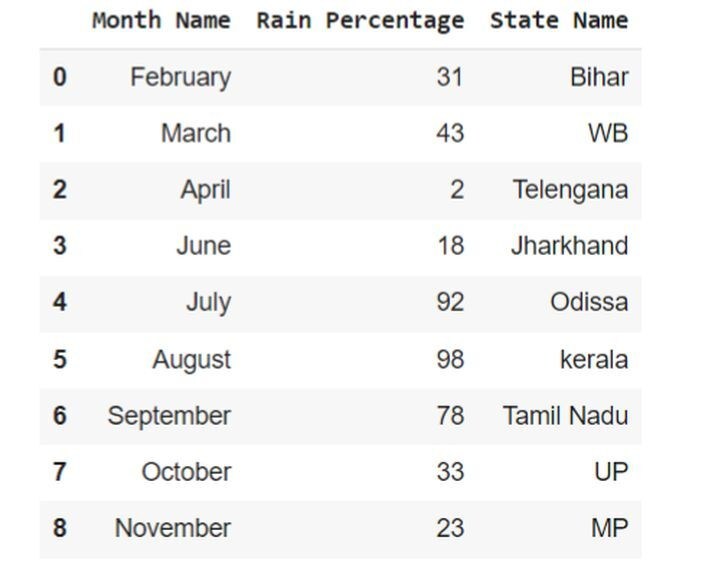## Example 2

In the following example, we will show how to skip a single row from the data. First, import the pandas module that helps to set the operation of reading the data. Take the pd as an object reference that will use to assign with read_csv. By using this built-in function it accepts two parameters- ‘demo.csv’(filename) and skiprows(set to value 1 that remove first row from the table).

#skip only single rows
import pandas as pd
df


## Output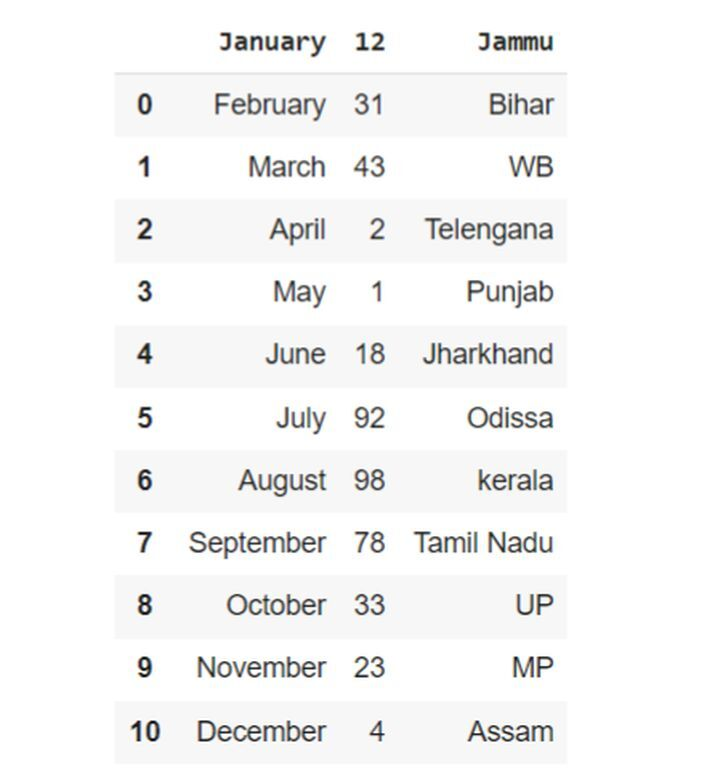## Example 3

In the following example, we will first import the pandas module that can be used to handle its object reference named pd. Next, we will store the value as built-in method read_csv with pd that accepts two parameters- ‘demo.csv’(filename) and skiprows( set the value for even condition). In the end, use the variable df to get the output.

# skip rows based on even condition
import pandas as pd
# print the months in even order
df


## Output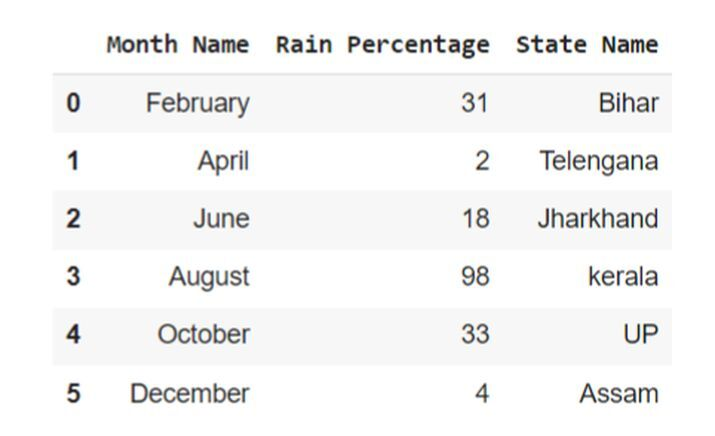## Example 4

In the following example, begin the program by importing the module named pandas. Then use the built-in method read_csv that sets two parameters- ‘demo.csv’(filename) and skiprows( set the value as lamda x:x>5 that sets only first five rows). Next, use the variable df to get the data of 5 rows.

# skip rows based on certain rows
import pandas as pd
df = pd.read_csv('demo.csv', skiprows= lambda x:x>5)
df


## Output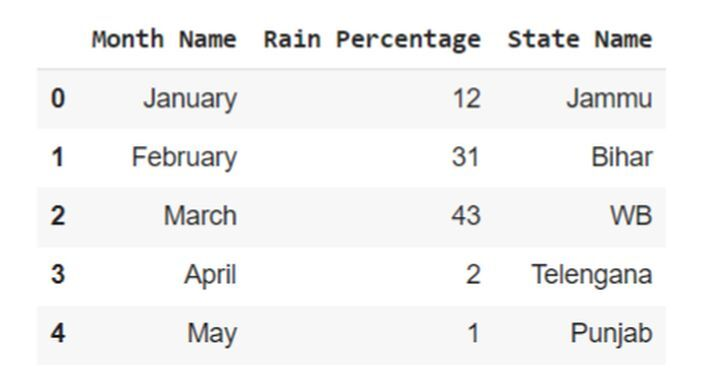## Example 5

In the following example, the program starts with pandas module and sets the object reference as pd. Then initialize the variable named df that stores the value by using the built-in method and it accepts three parameters- ‘demo.csv’(the name of the file), skiprows( set the value integer 2 and 10 in the list to delete the data from the table), and nrows( set the value as 10 that means only 10 rows will be available).

import pandas as pd
df


## Output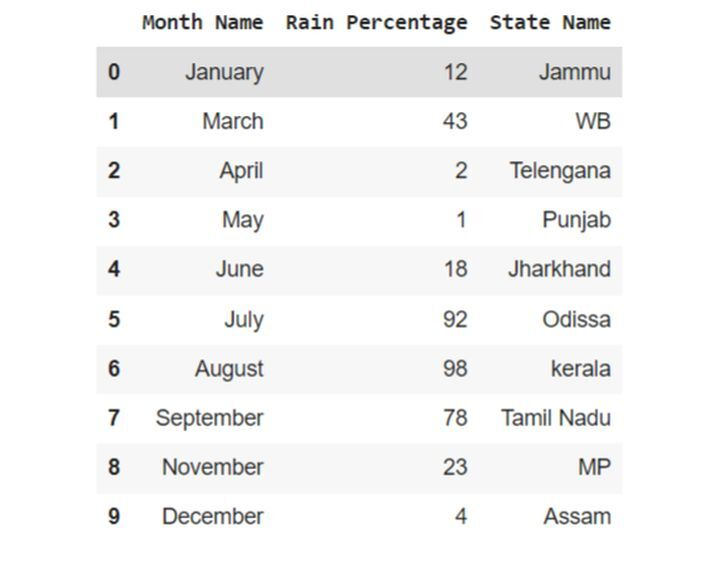## Conclusion

We discussed the concept of skiprows by applying various conditions to it. We used the skiprows condition for the skip of single rows, skipping of multiple rows, skipping rows based on even conditions, skipping rows based on certain conditions, and skipping the specific row from the CSV file.

Updated on: 17-Jul-2023

872 Views